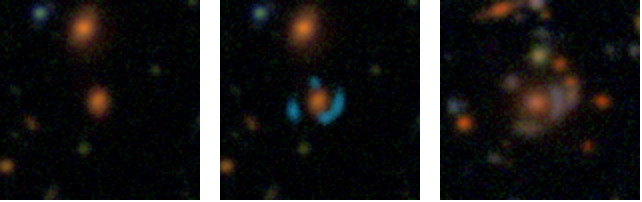## What is relaxed label shift?

Distribution shift is when the training and test data in supervised learning have different distributions.
Domain adaptation means training a classifier using only training data that gets good accuracy on test data under distribution shift. In our recent ICLR submission called RLSbench we loosely defined relax label shift as

The label marginal distribution, $$p(y)$$, can shift arbitrarily and the class conditionals, $$p(x\vert y)$$, can shift in seemingly natural ways.

This is admittedly, purposefully vague. But you can see it in our gravitational lens detection problem.
I have written in more detail about it here, but the setting fits the relaxed label shift definition. The basic idea is that we want to detect a rare phenomena called gravitational lenses, when very distant (old) light bends around a distant massive object like a galaxy and we can see it. In our study, we can simulate a very many realistic lenses over real astronomy images without lenses.Non-lens (left), simulated lens (center), real lens (right)

You can assume that there are no real lenses in our training set (we actually curate it). So because we get many simulated lenses, but there are very few real ones in the test set, the marginal label distribution $$p(y)$$ shifts ($$y=1$$ means it is a lens, $$y=0$$ means it is not). Also, because the simulations definitely look different than the real lenses (they aren’t THAT good), then $$p(x\vert y=1)$$ shifts. However, $$p(x\vert y=0)$$ is the same since the non-lenses in the training and test data are pulled from the set of survey images. Hence, we have relaxed label shift, and typical assumptions like covariate shift ($$p(y\vert x)$$ doesn’t shift) and label shift ($$p(x\vert y)$$ doesn’t shift) don’t hold.

## Consistency regularization works

The RLSbench work does A LOT, and it is all due to Saurabh Garg. One takeaway of many is that FixMatch works quite well for domain adaptation under many of our benchmark datasets, and with appropriate modification it can almost always outperform standard supervised learning. This is important because we wont have a chance to do model selection for our domain adaptation method without peeking at the test data, and so we can’t really choose it. We want it to be safe in the sense that it typically improves performance.

Consistency regularization is where two independently augmented samples of the same test image are encouraged to produce similar predictions. This needs test images, but that is fine because we still wont peek at the test labels - a setting called semi-supervised learning (SSL). The Pi-model algorithm directly uses consistency regularization. The idea is to take two random augmentations of the same sample data point x, and compute the squared difference of the model outputs for the augmented copies. We use $$\text{aug}, \widetilde{\text{aug}}$$ to denote two independent augmentations, which can be produced by selecting different randomization seeds. The unsupervised loss is then

$\ell_U(X) = \left\| p_\Theta(\text{aug}(X)) - p_\Theta(\widetilde{\text{aug}}(X))\right\|^2$

This unsupervised loss is added to the supervised loss (usually with data augmentation as well). The choice of stochastic augmentation function is up to the modeler and will often be domain specific. FixMatch and MixMatch employ both consistency regularization. MixMatch was originally proposed as a heuristic approach, and FixMatch was later derived as a more principled simplification of MixMatch and other related SSL methods. When we look at the relative performances of different SSL algorithms for detecting gravitational lenses, we see that consistency regularization does best. (This is combined with GAN and smart data augmentation.) For more details on the pipeline for detecting gravitational lenses see our recent preprint.